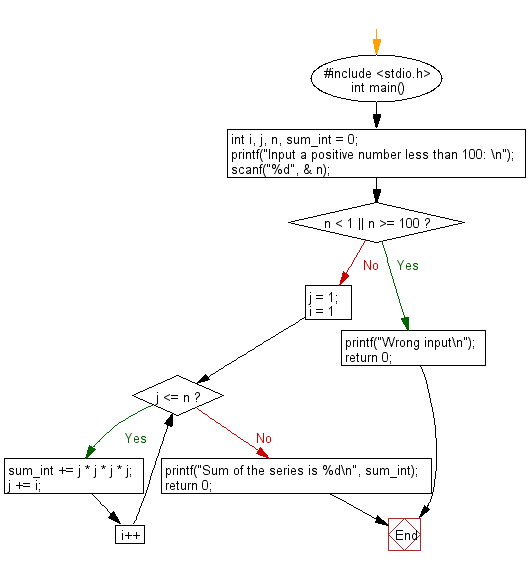﻿ C : Prints the sum of n positive integers less than 100

# C Exercises: Accepts a positive integer n less than 100 from the user and prints out the sum

## C Basic Declarations and Expressions: Exercise-63 with Solution

Write a C program that accepts a positive integer n less than 100 from the user. It prints out the sum of 14 + 24 + 44 + 74 + 114 + • • • + m4. In this case, m is less than or equal to n. Print an appropriate message.
Test data and expected output:
Input a positive number less than 100:
Sum of the series is 1024388

Sample Solution:

C Code:

``````#include <stdio.h>
int main() {
int i, j, n, sum_int = 0;

// Prompt user for input
printf("Input a positive number less than 100: \n");

scanf("%d", &n);

// Check if the input is valid
if (n < 1 || n >= 100) {
printf("Wrong input\n");
return 0;
}

j = 1;
for (i = 1; j <= n; i++) {
sum_int += j * j * j * j;
j += i;
}

// Display the result
printf("Sum of the series is %d\n", sum_int);

return 0;
}
``````

Sample Output:

```Input a positive number less than 100: 68
Sum of the series is 37361622
```

Flowchart:C programming Code Editor:

What is the difficulty level of this exercise?

Test your Programming skills with w3resource's quiz.

﻿